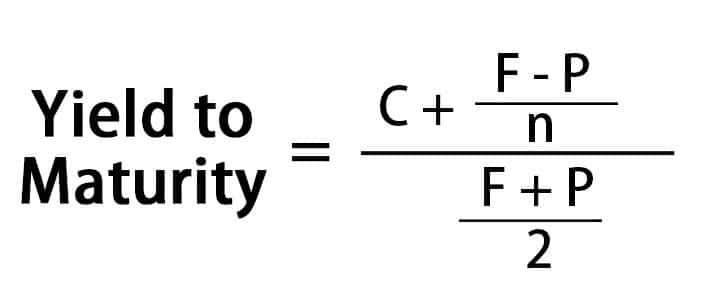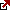# What is the Approximated Yield to Maturity YTM?

## Yield to maturity definition

So what is Yield to maturity? YTM or yield to maturity, redemption yield, or book yield of fixed- interest securities or bonds is basically the theoretical IRR (internal rate of return) that is earned by the investor that buys this bond at the present market price today, assuming that all the principal payments and coupons are mode at the schedule and is held until the maturity. In the examples below, we will calculate only approximated yield to maturity because true YTM is determined once the price matches security’s actual current market price.

Therefore Yield to maturity means the discounted rate wherein all the future cash flows such as principals and coupons from the bond are equal or same as the current rate of this bond. Yield to Maturity is mostly given at the terms of A.P.R (Annual Percentage Rate). It should be noted while doing this, often the market convections are followed. For several markets like gilts, the convention is to quote annualized yields along with the semi-annual compounding. (For reference, check compound interest). See the example below to get an idea.
The annual yield at 10.25% is always quoted as ten percentage; this is because 1.05% * 1.05% equals 1.1025 & 2*5 is equals 10.

Main assumptions

Following are the list of main assumptions that are used concerning convection yield measures:

All the principal payments and coupons are made on the schedule. Bond held till maturity. Yield to maturity always remains a single interest rate, which equates the present value of the bond’s cash flows to the price. One common misconception everyone has is the coupons should be reinvested at the yield to maturity. Well, this is not true. The actual paper says that this is a common misconception in the financial literature. The fact is coupon reinvestment is absolutely not required to hold the yield to maturity formula. According to Fabozzi, the yield to maturity process tells very little on potential yield, which an investment realizes if it is held till maturity. Without making allowance for the tax paid through the investor on return, the yield is usually quoted; hence, it is known as the gross redemption yield. Further, it does not make an allowance for the costs incurred by the seller or purchaser.

The coupon rate versus yield to maturity & parity

When an investor is looking for available options for his bond investment, he will mainly review two types of information. Yes, you got it right; it is the YTM (yield to maturity) and coupon rate. The bonds are mainly fixed-income investments used by investors in savings accounts and retirement account. It’s low-risk security that has a higher rate of returns compared to savings accounts.

Generally, an investor of a bond decides based on the instrument’s YTM rather than the coupon rate.

Here are some bullet points to let you understand the relation between the coupon rate and the YTM.

When the coupon rate of a bond remains lesser than the YTM, this means that the bond is being sold at a discount. When the coupon rate of a bond remains greater than the YTM, this means the bond is being sold at a premium rate. When the coupon rate of a bond remains the same as the YTM, this means the bond is being sold at par.

Special Considerations

NOTE that the coupon payment for a bond investor is the main source of profit. The YTM calculation incorporates potential losses or gains generated through the changes in market price. The changes in the market price generate the potential loss or gain of the bond trader.

When an investor buys a bond at face value or par value, the YTM automatically equals the coupon rate. When the investor buys the bond at a discount, the YTM gets higher than the coupon rate. When the investor purchases the bond at a premium, the YTM will be lower than the coupon rate.

Yield to maturity represents the average return of the bond over the bond’s remaining lifetime. A single discount rate is applied to future payments, thereby creating a value that equals the price of the bond. This way, time till the maturity, current price, coupon rate & the difference between the price and the face value will be considered.

## The formula for yield to maturity

Approximate yield to maturity formula

The price of a bond equals to present value of future cash flows. See the formula below:Here:

P= Bond Price

n- no. of periods

r- the rate of return

C= Coupon payment

t- Maturity period to receive the payment

F- maturity value

(Note: Approximated yield to maturity is because true value uses only real market prices in the calculation. )

By using financial software or calculate, the investor can calculate the percentage rate that can equal the present bond’s cash flow to today’s selling price. To get a brief, let us assume that you are the owner of a company X bond with thousand dollars par value & a coupon of 5%which will mature in 3 years. If the company is selling it for nine eighty dollars, using the formula, you can calculate that its YTM will be 2.87%.

The variants of YTM

Similar to the nature of bonds, which have variant characteristics, YTM too has some variants:

YTC or Yield to call: When the bond can be callable that is repurchased by the issuer before its maturity, the market looks to the YTC, which is the same calculation as YTM, assuming the bond would be called. Therefore cash flow is shortened. YTP or Yield to put: same as the YTC, but here the holder of the bond has an option to sell back the bond to the issuer at a fixed price at a specific date. YTW or Yield to worst: when the bond is exchangeable, puttable, or callable or has other types of features, YTW is considered as the lowest yield of YTM, YTP, YTC & others.

Bonds with coupon-bearing

For multiple coupon bonds, generally, you can’t solve the yield algebraically based on price. Newton’s method, a certified numerical technique of root-finding, is used to calculate the approximate yield. This explains that the future cash flow of today is equal to its bond price.

What is the importance of Yield to maturity?

Yield to maturity enables potential investors to understand and compare variants’ securities and returns to be expected from each of them. This further helps them to determine which securities they can consider for their portfolios. Also, investors need to know how changing market conditions can affect them; when there is a drop-in security price, the yield rises.

Yield to Maturity vs. Coupon Rate: What’s the Difference?

Many investors prefer to invest in bonds, which are fixed income securities, since they are a low-risk option for the investor, with little risk of default. The interest rate offered on bonds is usually slightly higher than the interest on the savings account. However, the interest rate for a specific bond will vary depending on the company issuing the bond. Since most bonds are low risk, they are preferred by retired people and others who do not wish to take risks. While choosing between the different bonds, they would like to find out Yield to Maturity vs. Coupon Rate: What’s the Difference?

Coupon rate

When a company or the government issues a bond, it will usually specify the bond issuer’s interest will pay the investor. The interest may be paid annually, monthly, quarterly, or every six months based on the bond terms. The coupon rate is the interest rate for the bond calculated annually by dividing the invested amount’s total interest. The coupon rate is linked to the market interest rate for debt. Most companies will issue bonds at par and specify the coupon rate. However, some companies may issue bonds with the zero-coupon rate at a discount to their face value.

Yield-to-Maturity

The yield of a bond to maturity, abbreviate as YTM, is the total profit which the investor in the bond will make till the maturity date. In addition to the income which the bond will pay periodically as specified by the bond issuer, the investor in the bonds can also make some additional profit by trading in bonds. The face or par value of bonds will vary depending on the market conditions. If the bond value interest rates are higher, the bond value will increase, and the bond value will decrease if the market interest rates are higher than the bond interest rates. In some cases, the bond issuer will also issue the bonds at a discount to their face value.

Comparison

Usually, the Coupon rate is applicable for both retail investors and bond traders since both will get paid interest periodically for their investment. However, bond traders who invest large amounts are interested in maximizing their profit to maximize the YTM of the bond. Depending on the bonds’ market value, they may sell or purchase bonds to maximize their YTM. If the investor purchases the bond at its face value and does not trade in it, the YTM is equal to the coupon rate. Another option for the investor to increase the YTM is by purchasing the bonds at a discount to the face value.

Yield

If the investor purchases the bonds at less than the face value, the YTM will be more than the bond’s coupon rate. Some companies which are finding it difficult to raise funds may issue the bonds at a discount. In some cases, the bonds may also be purchased at a discount in the bond trading market. The investor or trader may purchase the bonds at a premium to the face value in other cases. In this case, the YTM for the investor may be less than the bond’s coupon rate.

Latest posts by Fxigor (see all)

Fxigor

Igor has been a trader since 2007. Currently, Igor works for several prop trading companies. He is an expert in financial niche, long-term trading, and weekly technical levels. The primary field of Igor's research is the application of machine learning in algorithmic trading. Education: Computer Engineering and Ph.D. in machine learning. Igor regularly publishes trading-related videos on the Fxigor Youtube channel. To contact Igor write on: igor@forex.in.rs

Trade gold and silver. Visit the broker's page and start trading high liquidity spot metals - the most traded instruments in the world.Diversify your savings with a gold IRA.

Gold & silver portfolio-building assistance from product professionals.

If you want to trade stocks try: